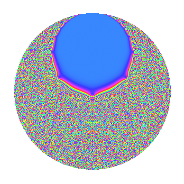# Properties

 Label 2.32.a.bLevel 2 Weight 32 Character orbit 2.a Self dual Yes Analytic conductor 12.175 Analytic rank 0 Dimension 2 CM No Inner twists 1

# Related objects

## Newspace parameters

 Level: $$N$$ = $$2$$ Weight: $$k$$ = $$32$$ Character orbit: $$[\chi]$$ = 2.a (trivial)

## Newform invariants

 Self dual: Yes Analytic conductor: $$12.1754265638$$ Analytic rank: $$0$$ Dimension: $$2$$ Coefficient field: $$\mathbb{Q}[x]/(x^{2} - \cdots)$$ Coefficient ring: $$\Z[a_1, a_2, a_3]$$ Coefficient ring index: $$2^{7}\cdot 3\cdot 5$$ Fricke sign: $$1$$ Sato-Tate group: $\mathrm{SU}(2)$

## $q$-expansion

Coefficients of the $$q$$-expansion are expressed in terms of $$\beta = 960\sqrt{987507049}$$. We also show the integral $$q$$-expansion of the trace form.

 $$f(q)$$ $$=$$ $$q$$ $$-32768 q^{2}$$ $$+ ( 8358252 - \beta ) q^{3}$$ $$+ 1073741824 q^{4}$$ $$+ ( -13381532850 - 3780 \beta ) q^{5}$$ $$+ ( -273883201536 + 32768 \beta ) q^{6}$$ $$+ ( -11751851055304 + 38502 \beta ) q^{7}$$ $$-35184372088832 q^{8}$$ $$+ ( 362273476569957 - 16716504 \beta ) q^{9}$$ $$+O(q^{10})$$ $$q$$ $$-32768 q^{2}$$ $$+(8358252 - \beta) q^{3}$$ $$+1073741824 q^{4}$$ $$+(-13381532850 - 3780 \beta) q^{5}$$ $$+(-273883201536 + 32768 \beta) q^{6}$$ $$+(-11751851055304 + 38502 \beta) q^{7}$$ $$-35184372088832 q^{8}$$ $$+(362273476569957 - 16716504 \beta) q^{9}$$ $$+(438486068428800 + 123863040 \beta) q^{10}$$ $$+(12575568739256292 - 209041371 \beta) q^{11}$$ $$+(8974604747931648 - 1073741824 \beta) q^{12}$$ $$+(94662172279854662 + 4745042748 \beta) q^{13}$$ $$+(385084655380201472 - 1261633536 \beta) q^{14}$$ $$+(3328280732528173800 - 18212659710 \beta) q^{15}$$ $$+1152921504606846976 q^{16}$$ $$+(460408414945337586 + 5927641128 \beta) q^{17}$$ $$+(-11870977280244350976 + 547766403072 \beta) q^{18}$$ $$+(-50043450866248766500 - 917688823029 \beta) q^{19}$$ $$+(-14368311490274918400 - 4058744094720 \beta) q^{20}$$ $$+(-$$$$13\!\cdots\!08$$$$+ 12073660473808 \beta) q^{21}$$ $$+(-$$$$41\!\cdots\!56$$$$+ 6849867644928 \beta) q^{22}$$ $$+($$$$25\!\cdots\!12$$$$- 57371978548206 \beta) q^{23}$$ $$+(-$$$$29\!\cdots\!64$$$$+ 35184372088832 \beta) q^{24}$$ $$+($$$$85\!\cdots\!75$$$$+ 101164388346000 \beta) q^{25}$$ $$+(-$$$$31\!\cdots\!16$$$$- 155485560766464 \beta) q^{26}$$ $$+($$$$13\!\cdots\!20$$$$+ 115679166722982 \beta) q^{27}$$ $$+(-$$$$12\!\cdots\!96$$$$+ 41341207707648 \beta) q^{28}$$ $$+(-$$$$19\!\cdots\!90$$$$- 912080882507508 \beta) q^{29}$$ $$+(-$$$$10\!\cdots\!00$$$$+ 596792433377280 \beta) q^{30}$$ $$+($$$$24\!\cdots\!92$$$$+ 3254688883162008 \beta) q^{31}$$ $$-$$$$37\!\cdots\!68$$$$q^{32}$$ $$+($$$$29\!\cdots\!84$$$$- 14322789196499784 \beta) q^{33}$$ $$+(-$$$$15\!\cdots\!48$$$$- 194236944482304 \beta) q^{34}$$ $$+($$$$24\!\cdots\!00$$$$+ 43906781211258420 \beta) q^{35}$$ $$+($$$$38\!\cdots\!68$$$$- 17949209495863296 \beta) q^{36}$$ $$+($$$$94\!\cdots\!26$$$$- 51326225439746292 \beta) q^{37}$$ $$+($$$$16\!\cdots\!00$$$$+ 30070827353014272 \beta) q^{38}$$ $$+(-$$$$35\!\cdots\!76$$$$- 55001909241298166 \beta) q^{39}$$ $$+($$$$47\!\cdots\!00$$$$+ 132996926495784960 \beta) q^{40}$$ $$+(-$$$$14\!\cdots\!18$$$$+ 104709028836970512 \beta) q^{41}$$ $$+($$$$43\!\cdots\!44$$$$- 395629706405740544 \beta) q^{42}$$ $$+(-$$$$14\!\cdots\!68$$$$+ 414868077394813917 \beta) q^{43}$$ $$+($$$$13\!\cdots\!08$$$$- 224456462989000704 \beta) q^{44}$$ $$+($$$$52\!\cdots\!50$$$$- 1145701294021281060 \beta) q^{45}$$ $$+(-$$$$83\!\cdots\!16$$$$+ 1879964993067614208 \beta) q^{46}$$ $$+($$$$66\!\cdots\!76$$$$+ 900502500303526212 \beta) q^{47}$$ $$+($$$$96\!\cdots\!52$$$$- 1152921504606846976 \beta) q^{48}$$ $$+(-$$$$18\!\cdots\!27$$$$- 904939538662629216 \beta) q^{49}$$ $$+(-$$$$27\!\cdots\!00$$$$- 3314954677321728000 \beta) q^{50}$$ $$+(-$$$$15\!\cdots\!28$$$$- 410863696631949330 \beta) q^{51}$$ $$+($$$$10\!\cdots\!88$$$$+ 5094950855195492352 \beta) q^{52}$$ $$+(-$$$$22\!\cdots\!18$$$$+ 16855972608155554188 \beta) q^{53}$$ $$+(-$$$$42\!\cdots\!60$$$$- 3790574935178674176 \beta) q^{54}$$ $$+($$$$55\!\cdots\!00$$$$- 44738355861343246410 \beta) q^{55}$$ $$+($$$$41\!\cdots\!28$$$$- 1354668694164209664 \beta) q^{56}$$ $$+($$$$41\!\cdots\!00$$$$+ 42373176425788981192 \beta) q^{57}$$ $$+($$$$64\!\cdots\!20$$$$+ 29887066358006022144 \beta) q^{58}$$ $$+(-$$$$12\!\cdots\!80$$$$- 15588326499987499191 \beta) q^{59}$$ $$+($$$$35\!\cdots\!00$$$$- 19555694456906711040 \beta) q^{60}$$ $$+($$$$36\!\cdots\!82$$$$- 22159967545724485284 \beta) q^{61}$$ $$+(-$$$$81\!\cdots\!56$$$$-$$$$10\!\cdots\!44$$$$\beta) q^{62}$$ $$+(-$$$$48\!\cdots\!28$$$$+$$$$21\!\cdots\!30$$$$\beta) q^{63}$$ $$+$$$$12\!\cdots\!24$$$$q^{64}$$ $$+(-$$$$17\!\cdots\!00$$$$-$$$$42\!\cdots\!60$$$$\beta) q^{65}$$ $$+(-$$$$96\!\cdots\!12$$$$+$$$$46\!\cdots\!12$$$$\beta) q^{66}$$ $$+($$$$10\!\cdots\!96$$$$+$$$$30\!\cdots\!11$$$$\beta) q^{67}$$ $$+($$$$49\!\cdots\!64$$$$+ 6364756196796137472 \beta) q^{68}$$ $$+($$$$54\!\cdots\!24$$$$-$$$$73\!\cdots\!24$$$$\beta) q^{69}$$ $$+(-$$$$81\!\cdots\!00$$$$-$$$$14\!\cdots\!60$$$$\beta) q^{70}$$ $$+($$$$18\!\cdots\!92$$$$+$$$$14\!\cdots\!66$$$$\beta) q^{71}$$ $$+(-$$$$12\!\cdots\!24$$$$+$$$$58\!\cdots\!28$$$$\beta) q^{72}$$ $$+($$$$82\!\cdots\!42$$$$+$$$$19\!\cdots\!08$$$$\beta) q^{73}$$ $$+(-$$$$30\!\cdots\!68$$$$+$$$$16\!\cdots\!56$$$$\beta) q^{74}$$ $$+(-$$$$20\!\cdots\!00$$$$-$$$$76\!\cdots\!75$$$$\beta) q^{75}$$ $$+(-$$$$53\!\cdots\!00$$$$-$$$$98\!\cdots\!96$$$$\beta) q^{76}$$ $$+(-$$$$15\!\cdots\!68$$$$+$$$$29\!\cdots\!68$$$$\beta) q^{77}$$ $$+($$$$11\!\cdots\!68$$$$+$$$$18\!\cdots\!88$$$$\beta) q^{78}$$ $$+(-$$$$21\!\cdots\!60$$$$+$$$$70\!\cdots\!76$$$$\beta) q^{79}$$ $$+(-$$$$15\!\cdots\!00$$$$-$$$$43\!\cdots\!80$$$$\beta) q^{80}$$ $$+(-$$$$21\!\cdots\!39$$$$-$$$$17\!\cdots\!68$$$$\beta) q^{81}$$ $$+($$$$47\!\cdots\!24$$$$-$$$$34\!\cdots\!16$$$$\beta) q^{82}$$ $$+($$$$20\!\cdots\!12$$$$-$$$$69\!\cdots\!65$$$$\beta) q^{83}$$ $$+(-$$$$14\!\cdots\!92$$$$+$$$$12\!\cdots\!92$$$$\beta) q^{84}$$ $$+(-$$$$26\!\cdots\!00$$$$-$$$$18\!\cdots\!80$$$$\beta) q^{85}$$ $$+($$$$47\!\cdots\!24$$$$-$$$$13\!\cdots\!56$$$$\beta) q^{86}$$ $$+($$$$66\!\cdots\!20$$$$+$$$$12\!\cdots\!74$$$$\beta) q^{87}$$ $$+(-$$$$44\!\cdots\!44$$$$+$$$$73\!\cdots\!72$$$$\beta) q^{88}$$ $$+($$$$26\!\cdots\!10$$$$+$$$$42\!\cdots\!80$$$$\beta) q^{89}$$ $$+(-$$$$17\!\cdots\!00$$$$+$$$$37\!\cdots\!80$$$$\beta) q^{90}$$ $$+(-$$$$94\!\cdots\!48$$$$-$$$$52\!\cdots\!68$$$$\beta) q^{91}$$ $$+($$$$27\!\cdots\!88$$$$-$$$$61\!\cdots\!44$$$$\beta) q^{92}$$ $$+(-$$$$27\!\cdots\!16$$$$+$$$$23\!\cdots\!24$$$$\beta) q^{93}$$ $$+(-$$$$21\!\cdots\!68$$$$-$$$$29\!\cdots\!16$$$$\beta) q^{94}$$ $$+($$$$38\!\cdots\!00$$$$+$$$$20\!\cdots\!50$$$$\beta) q^{95}$$ $$+(-$$$$31\!\cdots\!36$$$$+$$$$37\!\cdots\!68$$$$\beta) q^{96}$$ $$+($$$$46\!\cdots\!86$$$$-$$$$87\!\cdots\!80$$$$\beta) q^{97}$$ $$+($$$$60\!\cdots\!36$$$$+$$$$29\!\cdots\!88$$$$\beta) q^{98}$$ $$+($$$$77\!\cdots\!44$$$$-$$$$28\!\cdots\!15$$$$\beta) q^{99}$$ $$+O(q^{100})$$ $$\operatorname{Tr}(f)(q)$$ $$=$$ $$2q$$ $$\mathstrut -\mathstrut 65536q^{2}$$ $$\mathstrut +\mathstrut 16716504q^{3}$$ $$\mathstrut +\mathstrut 2147483648q^{4}$$ $$\mathstrut -\mathstrut 26763065700q^{5}$$ $$\mathstrut -\mathstrut 547766403072q^{6}$$ $$\mathstrut -\mathstrut 23503702110608q^{7}$$ $$\mathstrut -\mathstrut 70368744177664q^{8}$$ $$\mathstrut +\mathstrut 724546953139914q^{9}$$ $$\mathstrut +\mathstrut O(q^{10})$$ $$2q$$ $$\mathstrut -\mathstrut 65536q^{2}$$ $$\mathstrut +\mathstrut 16716504q^{3}$$ $$\mathstrut +\mathstrut 2147483648q^{4}$$ $$\mathstrut -\mathstrut 26763065700q^{5}$$ $$\mathstrut -\mathstrut 547766403072q^{6}$$ $$\mathstrut -\mathstrut 23503702110608q^{7}$$ $$\mathstrut -\mathstrut 70368744177664q^{8}$$ $$\mathstrut +\mathstrut 724546953139914q^{9}$$ $$\mathstrut +\mathstrut 876972136857600q^{10}$$ $$\mathstrut +\mathstrut 25151137478512584q^{11}$$ $$\mathstrut +\mathstrut 17949209495863296q^{12}$$ $$\mathstrut +\mathstrut 189324344559709324q^{13}$$ $$\mathstrut +\mathstrut 770169310760402944q^{14}$$ $$\mathstrut +\mathstrut 6656561465056347600q^{15}$$ $$\mathstrut +\mathstrut 2305843009213693952q^{16}$$ $$\mathstrut +\mathstrut 920816829890675172q^{17}$$ $$\mathstrut -\mathstrut 23741954560488701952q^{18}$$ $$\mathstrut -\mathstrut$$$$10\!\cdots\!00$$$$q^{19}$$ $$\mathstrut -\mathstrut 28736622980549836800q^{20}$$ $$\mathstrut -\mathstrut$$$$26\!\cdots\!16$$$$q^{21}$$ $$\mathstrut -\mathstrut$$$$82\!\cdots\!12$$$$q^{22}$$ $$\mathstrut +\mathstrut$$$$51\!\cdots\!24$$$$q^{23}$$ $$\mathstrut -\mathstrut$$$$58\!\cdots\!28$$$$q^{24}$$ $$\mathstrut +\mathstrut$$$$17\!\cdots\!50$$$$q^{25}$$ $$\mathstrut -\mathstrut$$$$62\!\cdots\!32$$$$q^{26}$$ $$\mathstrut +\mathstrut$$$$26\!\cdots\!40$$$$q^{27}$$ $$\mathstrut -\mathstrut$$$$25\!\cdots\!92$$$$q^{28}$$ $$\mathstrut -\mathstrut$$$$39\!\cdots\!80$$$$q^{29}$$ $$\mathstrut -\mathstrut$$$$21\!\cdots\!00$$$$q^{30}$$ $$\mathstrut +\mathstrut$$$$49\!\cdots\!84$$$$q^{31}$$ $$\mathstrut -\mathstrut$$$$75\!\cdots\!36$$$$q^{32}$$ $$\mathstrut +\mathstrut$$$$59\!\cdots\!68$$$$q^{33}$$ $$\mathstrut -\mathstrut$$$$30\!\cdots\!96$$$$q^{34}$$ $$\mathstrut +\mathstrut$$$$49\!\cdots\!00$$$$q^{35}$$ $$\mathstrut +\mathstrut$$$$77\!\cdots\!36$$$$q^{36}$$ $$\mathstrut +\mathstrut$$$$18\!\cdots\!52$$$$q^{37}$$ $$\mathstrut +\mathstrut$$$$32\!\cdots\!00$$$$q^{38}$$ $$\mathstrut -\mathstrut$$$$70\!\cdots\!52$$$$q^{39}$$ $$\mathstrut +\mathstrut$$$$94\!\cdots\!00$$$$q^{40}$$ $$\mathstrut -\mathstrut$$$$29\!\cdots\!36$$$$q^{41}$$ $$\mathstrut +\mathstrut$$$$87\!\cdots\!88$$$$q^{42}$$ $$\mathstrut -\mathstrut$$$$29\!\cdots\!36$$$$q^{43}$$ $$\mathstrut +\mathstrut$$$$27\!\cdots\!16$$$$q^{44}$$ $$\mathstrut +\mathstrut$$$$10\!\cdots\!00$$$$q^{45}$$ $$\mathstrut -\mathstrut$$$$16\!\cdots\!32$$$$q^{46}$$ $$\mathstrut +\mathstrut$$$$13\!\cdots\!52$$$$q^{47}$$ $$\mathstrut +\mathstrut$$$$19\!\cdots\!04$$$$q^{48}$$ $$\mathstrut -\mathstrut$$$$36\!\cdots\!54$$$$q^{49}$$ $$\mathstrut -\mathstrut$$$$55\!\cdots\!00$$$$q^{50}$$ $$\mathstrut -\mathstrut$$$$30\!\cdots\!56$$$$q^{51}$$ $$\mathstrut +\mathstrut$$$$20\!\cdots\!76$$$$q^{52}$$ $$\mathstrut -\mathstrut$$$$45\!\cdots\!36$$$$q^{53}$$ $$\mathstrut -\mathstrut$$$$85\!\cdots\!20$$$$q^{54}$$ $$\mathstrut +\mathstrut$$$$11\!\cdots\!00$$$$q^{55}$$ $$\mathstrut +\mathstrut$$$$82\!\cdots\!56$$$$q^{56}$$ $$\mathstrut +\mathstrut$$$$83\!\cdots\!00$$$$q^{57}$$ $$\mathstrut +\mathstrut$$$$12\!\cdots\!40$$$$q^{58}$$ $$\mathstrut -\mathstrut$$$$25\!\cdots\!60$$$$q^{59}$$ $$\mathstrut +\mathstrut$$$$71\!\cdots\!00$$$$q^{60}$$ $$\mathstrut +\mathstrut$$$$73\!\cdots\!64$$$$q^{61}$$ $$\mathstrut -\mathstrut$$$$16\!\cdots\!12$$$$q^{62}$$ $$\mathstrut -\mathstrut$$$$96\!\cdots\!56$$$$q^{63}$$ $$\mathstrut +\mathstrut$$$$24\!\cdots\!48$$$$q^{64}$$ $$\mathstrut -\mathstrut$$$$35\!\cdots\!00$$$$q^{65}$$ $$\mathstrut -\mathstrut$$$$19\!\cdots\!24$$$$q^{66}$$ $$\mathstrut +\mathstrut$$$$21\!\cdots\!92$$$$q^{67}$$ $$\mathstrut +\mathstrut$$$$98\!\cdots\!28$$$$q^{68}$$ $$\mathstrut +\mathstrut$$$$10\!\cdots\!48$$$$q^{69}$$ $$\mathstrut -\mathstrut$$$$16\!\cdots\!00$$$$q^{70}$$ $$\mathstrut +\mathstrut$$$$36\!\cdots\!84$$$$q^{71}$$ $$\mathstrut -\mathstrut$$$$25\!\cdots\!48$$$$q^{72}$$ $$\mathstrut +\mathstrut$$$$16\!\cdots\!84$$$$q^{73}$$ $$\mathstrut -\mathstrut$$$$61\!\cdots\!36$$$$q^{74}$$ $$\mathstrut -\mathstrut$$$$41\!\cdots\!00$$$$q^{75}$$ $$\mathstrut -\mathstrut$$$$10\!\cdots\!00$$$$q^{76}$$ $$\mathstrut -\mathstrut$$$$31\!\cdots\!36$$$$q^{77}$$ $$\mathstrut +\mathstrut$$$$23\!\cdots\!36$$$$q^{78}$$ $$\mathstrut -\mathstrut$$$$43\!\cdots\!20$$$$q^{79}$$ $$\mathstrut -\mathstrut$$$$30\!\cdots\!00$$$$q^{80}$$ $$\mathstrut -\mathstrut$$$$43\!\cdots\!78$$$$q^{81}$$ $$\mathstrut +\mathstrut$$$$95\!\cdots\!48$$$$q^{82}$$ $$\mathstrut +\mathstrut$$$$41\!\cdots\!24$$$$q^{83}$$ $$\mathstrut -\mathstrut$$$$28\!\cdots\!84$$$$q^{84}$$ $$\mathstrut -\mathstrut$$$$53\!\cdots\!00$$$$q^{85}$$ $$\mathstrut +\mathstrut$$$$95\!\cdots\!48$$$$q^{86}$$ $$\mathstrut +\mathstrut$$$$13\!\cdots\!40$$$$q^{87}$$ $$\mathstrut -\mathstrut$$$$88\!\cdots\!88$$$$q^{88}$$ $$\mathstrut +\mathstrut$$$$52\!\cdots\!20$$$$q^{89}$$ $$\mathstrut -\mathstrut$$$$34\!\cdots\!00$$$$q^{90}$$ $$\mathstrut -\mathstrut$$$$18\!\cdots\!96$$$$q^{91}$$ $$\mathstrut +\mathstrut$$$$54\!\cdots\!76$$$$q^{92}$$ $$\mathstrut -\mathstrut$$$$55\!\cdots\!32$$$$q^{93}$$ $$\mathstrut -\mathstrut$$$$43\!\cdots\!36$$$$q^{94}$$ $$\mathstrut +\mathstrut$$$$76\!\cdots\!00$$$$q^{95}$$ $$\mathstrut -\mathstrut$$$$63\!\cdots\!72$$$$q^{96}$$ $$\mathstrut +\mathstrut$$$$93\!\cdots\!72$$$$q^{97}$$ $$\mathstrut +\mathstrut$$$$12\!\cdots\!72$$$$q^{98}$$ $$\mathstrut +\mathstrut$$$$15\!\cdots\!88$$$$q^{99}$$ $$\mathstrut +\mathstrut O(q^{100})$$

## Embeddings

For each embedding $$\iota_m$$ of the coefficient field, the values $$\iota_m(a_n)$$ are shown below.

For more information on an embedded modular form you can click on its label.

Label $$\iota_m(\nu)$$ $$a_{2}$$ $$a_{3}$$ $$a_{4}$$ $$a_{5}$$ $$a_{6}$$ $$a_{7}$$ $$a_{8}$$ $$a_{9}$$ $$a_{10}$$
1.1
 15712.8 −15711.8
−32768.0 −2.18094e7 1.07374e9 −1.27415e11 7.14650e11 −1.05903e13 −3.51844e13 −1.42024e14 4.17514e15
1.2 −32768.0 3.85259e7 1.07374e9 1.00652e11 −1.26242e12 −1.29134e13 −3.51844e13 8.66571e14 −3.29817e15
 $$n$$: e.g. 2-40 or 990-1000 Significant digits: Format: Complex embeddings Normalized embeddings Satake parameters Satake angles

## Inner twists

This newform does not admit any (nontrivial) inner twists.

## Atkin-Lehner signs

$$p$$ Sign
$$2$$ $$1$$

## Hecke kernels

This newform can be constructed as the kernel of the linear operator $$T_{3}^{2}$$ $$\mathstrut -\mathstrut 16716504 T_{3}$$ $$\mathstrut -\mathstrut$$$$84\!\cdots\!96$$ acting on $$S_{32}^{\mathrm{new}}(\Gamma_0(2))$$.